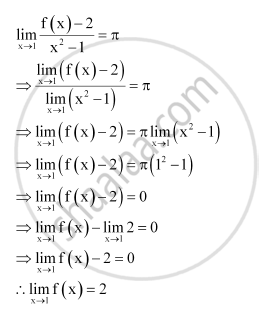CBSE (Commerce) Class 11CBSE
Share
Notifications

View all notifications
Books Shortlist
Your shortlist is empty

# Solution for If the Function F(X) Satisfies Lim_(X -> 1) (F(X) - 2)/(X^2 - 1) = Pi, Evaluate Lim_(X -> 1) F(X) - CBSE (Commerce) Class 11 - Mathematics

Login
Create free account

Forgot password?
ConceptLimits Limits of Polynomials and Rational Functions

#### Question

If the function f(x) satisfies lim_(x -> 1) (f(x) - 2)/(x^2 - 1) = pi, evaluate lim_(x -> 1) f(x)

#### SolutionIs there an error in this question or solution?

#### APPEARS IN

NCERT Solution for Mathematics Textbook for Class 11 (2013 to Current)
Chapter 13: Limits and Derivatives
Q: 31 | Page no. 303
Solution If the Function F(X) Satisfies Lim_(X -> 1) (F(X) - 2)/(X^2 - 1) = Pi, Evaluate Lim_(X -> 1) F(X) Concept: Limits - Limits of Polynomials and Rational Functions.
S